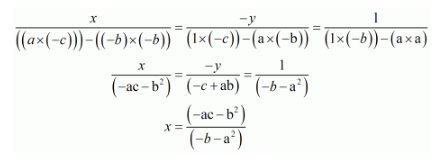# Solve each of the following systems of equations by the method of cross-multiplication :Question:

Solve each of the following systems of equations by the method of cross-multiplication :

$x+a y=b$

$a x-b y=c$

Solution:

GIVEN:

$x+a y=b$

$a x-b y=c$

To find: The solution of the systems of equation by the method of cross-multiplication:

Here we have the pair of simultaneous equation

$x+a y-b=0$

$a x-b y-c=0$

By cross multiplication method we get$x=\frac{\left(a c+b^{2}\right)}{\left(b+a^{2}\right)}$, and $y=\frac{-(-c+a b)}{\left(-b-a^{2}\right)} y=\frac{(a b-c)}{\left(a^{2}+b\right)}$

Hence we get the value of $x=\frac{\left(\mathrm{ac}+\mathrm{b}^{2}\right)}{\left(b+\mathrm{a}^{2}\right)}$ and $\mathrm{y}=\frac{(\mathrm{ab}-\mathrm{c})}{\left(\mathrm{a}^{2}+b\right)}$